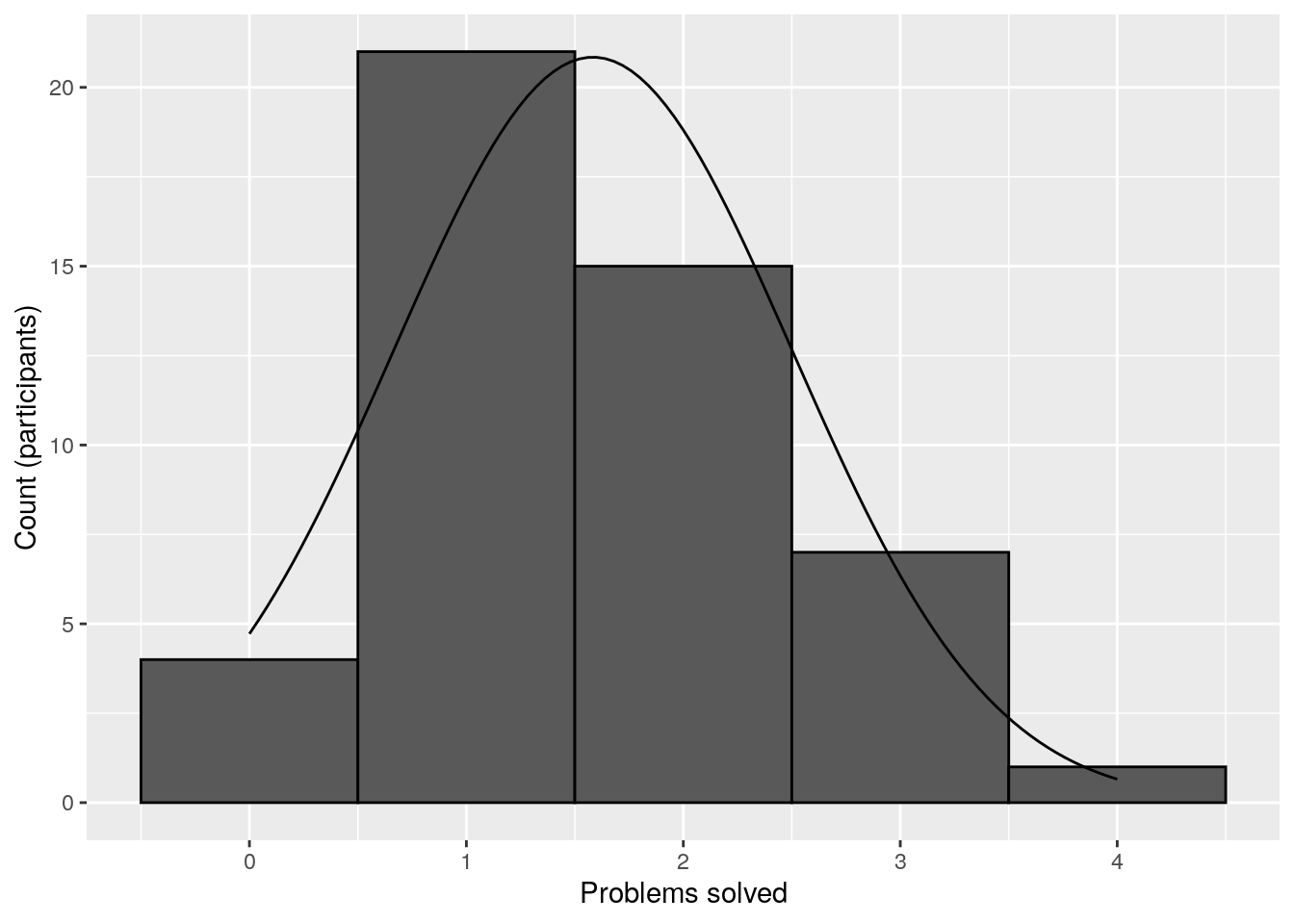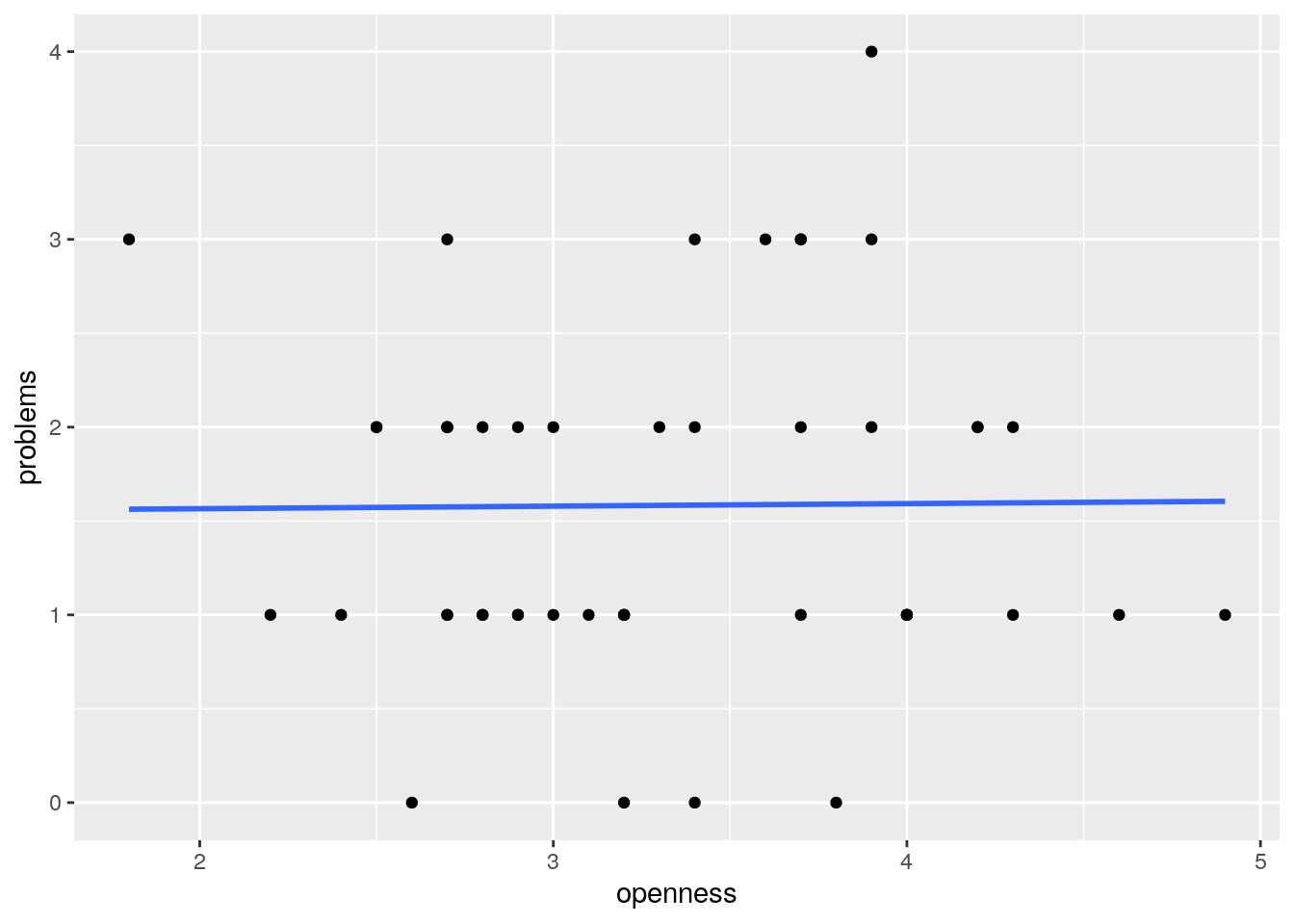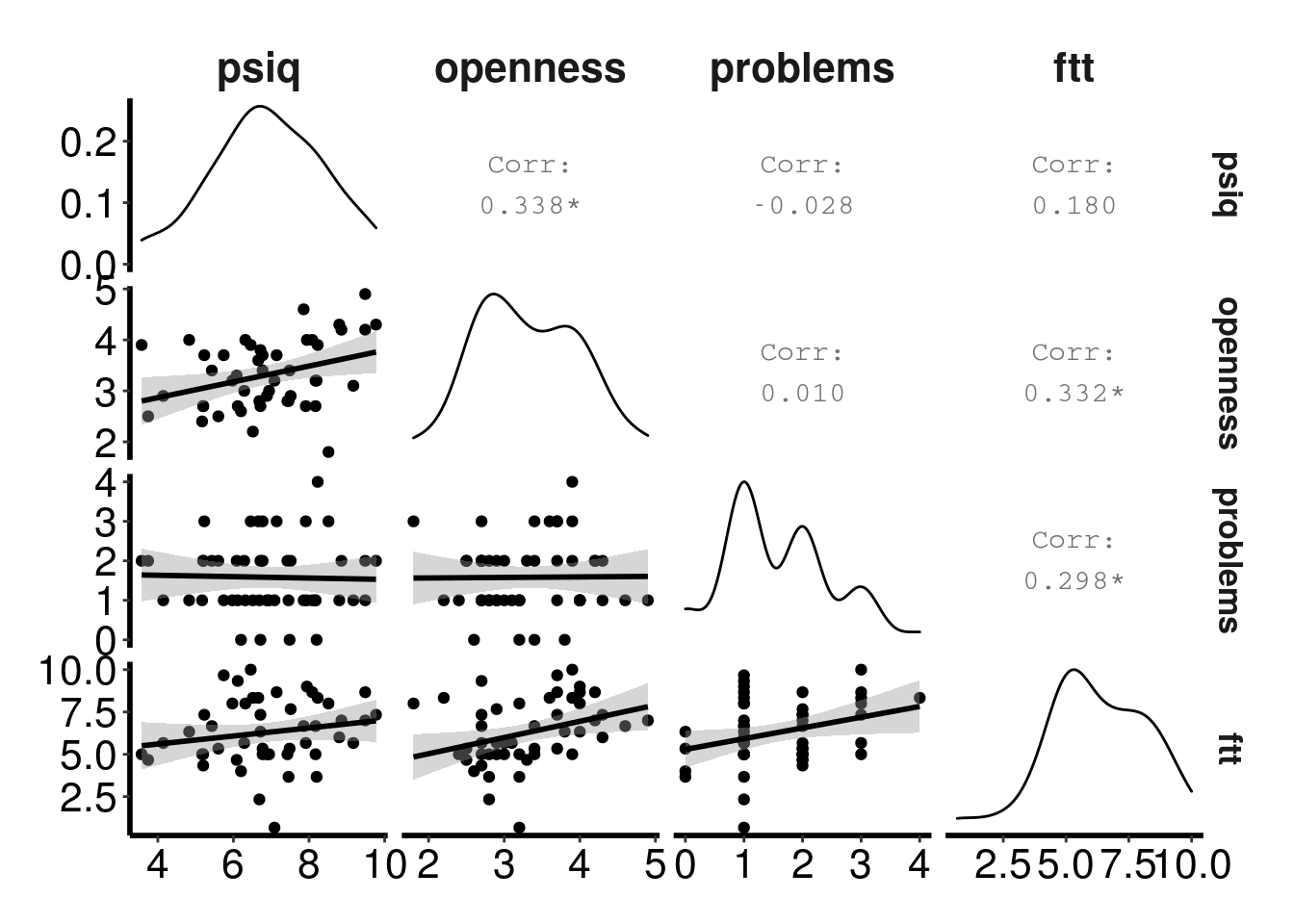## Before you start

This is an advanced worksheet, which assumes you have completed the Absolute Beginners’ Guide to R course, the Research Methods in Practice (Quantitative section) course, and the Intermediate Guide to R course.

## Introduction

This worksheet describes a full analysis pipeline for an undergraduate student dissertation which explored relationships between personality, imagery and creative problem solving. Forty-eight students were tested to address three hypotheses. First, the researchers predicted that participants with more open personality types would be better at solving a selection of problems requiring creative solutions. Second, they predicated that participants with more vivid mental imagery would be better at solving the problems. Third, they predicted a relationship between divergent thinking and an ability to solve the problems.

Personality was measured using a simplified version of Costa and McCrae’s (1992) “big five” personality questionnaire. Mental imagery was measured using the PsiQ Plymouth Sensory Imagery Questionnaire (Andrade et al., 2013). Divergent thinking was measured using a ‘flexible thinking task’, which measured fluency, flexibility and originality. The problems requiring creative solutions were taken from May (1987).

Open the rminr-data project we used previously.

Ensure you have the latest files by asking git to “pull” the repository. Select the Git tab, which is located in the row of tabs which includes the Environment tab. Click the Pull button with a downward pointing arrow. A window will open showing the files which have been pulled from the repository. Close the Git pull window. The case-studies folder should contain the folder jon-may.

Next, create a new, empty R script and save it in the rminr-data folder as cs-jon-may.R. Put all the commands from this worksheet into this file, and run them from there. Save your script regularly.

rm(list = ls()) # clear the environment
library(tidyverse)

Explanation of commands:

We clear the workspace, and load tidyverse package, then read the four data files.

## Preprocessing

### Problems

Problem booklet with 7 problems (solved=1, not=0, total=n solved)

problems <- read_csv('case-studies/jon-may/problems.csv')
Parsed with column specification:
cols(
subj = col_double(),
bridges = col_double(),
coins = col_double(),
greengrocer = col_double(),
wolves = col_double(),
cards = col_double(),
gorge = col_double(),
dots = col_double()
)
solved <- problems %>%
rowwise() %>%
mutate(problems = sum(c_across(bridges:dots))) %>%
select(subj, problems)

### OCEAN

Big5 OCEAN questionnaire 50 items answered 1 to 5 (includes a data entry error)

ocean   <- read_csv('case-studies/jon-may/big5.csv')
Parsed with column specification:
cols(
.default = col_double()
)
See spec(...) for full column specifications.
#big5    <- read_csv('case-studies/jon-may/big5_total.csv', col_types = 'fiiiii')

scaleIDs <- oceankey$ScaleID # make a vector of the new variable names names(scaleIDs) <- oceankey$ItemID           # name each new name with the old name so it can be looked-up

ocean.scales<-ocean %>%
pivot_longer(S1:S50,names_to='Item', values_to='Value') %>%     # put the 50 items into a column
mutate(ScaleID=scaleIDs[Item])%>%                               # lookup the new names from the vector
select(-Item) %>%                                               # drop the columns we don't need
mutate(Value=ifelse(Value>5,NA,ifelse(Value<1, NA, Value))) %>% # screen for absurd values
mutate(Value=ifelse(grepl("r",ScaleID), 6-Value, Value)) %>%    # reverse score items containg 'r' ...
mutate(ScaleID = sub("r", "", ScaleID)) %>%                     # ... and remove the 'r'
pivot_wider(names_from="ScaleID", values_from="Value") %>%      # make the data wide again
select(subj,sort(names(.))) %>%                                 # sort the columns by the new names
rowwise() %>%                                                   # for each participant...
mutate(openness=mean(c_across(o01:o10), na.rm = TRUE),          # ... mean of 10 items in each scale
conscientiousness=mean(c_across(C01:C10), na.rm = TRUE),
extraversion=mean(c_across(E01:E10), na.rm = TRUE),
agreeableness=mean(c_across(A01:A10), na.rm = TRUE),
neuroticism=mean(c_across(N01:N10), na.rm = TRUE)) %>%
select(subj, openness, conscientiousness, extraversion, agreeableness, neuroticism)

### Flexible thinking test

• We don’t have raw data for the ‘Flexible Thinking Test’ (overall scores mean of ftt1-3)
ftt <- read_csv('case-studies/jon-may/ftt.csv') %>%
rowwise() %>%
mutate(ftt = mean(c_across(ftt1:ftt3))) %>%
select(subj, ftt)
Parsed with column specification:
cols(
subj = col_double(),
ftt1 = col_double(),
ftt2 = col_double(),
ftt3 = col_double()
)
• the 35 PsiQ vividness of imagery items - 5 of each of 7 modalities (0 not at all to 10 as vivid as real life) (score = mean of all items)
psiq <- read_csv('case-studies/jon-may/psiq.csv') %>%
rowwise() %>%
mutate(psiq = mean(c_across(2:36))) %>%
select(subj, psiq)
Parsed with column specification:
cols(
.default = col_double()
)
See spec(...) for full column specifications.
data <- full_join(ocean.scales, solved, by = 'subj') %>%
full_join(ftt, by = 'subj') %>%
full_join(psiq, by = 'subj')

## Mean, Standard deviation, skewness, kurtosis for all independent variables (n=48)

The psych library has a useful function describe to obtain descriptive statistics.

library(psych)

Attaching package: 'psych'
The following objects are masked from 'package:ggplot2':

%+%, alpha
describe(data)
                  vars  n  mean    sd median trimmed   mad  min   max range
subj                 1 48 24.50 14.00  24.50   24.50 17.79 1.00 48.00 47.00
openness             2 48  3.31  0.68   3.20    3.30  0.74 1.80  4.90  3.10
conscientiousness    3 48  3.51  0.72   3.50    3.51  0.74 2.20  4.90  2.70
extraversion         4 48  3.15  0.79   3.30    3.15  0.82 1.30  4.90  3.60
agreeableness        5 48  4.36  0.46   4.40    4.38  0.58 3.30  5.00  1.70
neuroticism          6 48  2.60  0.74   2.50    2.58  0.89 1.30  4.80  3.50
problems             7 48  1.58  0.92   1.00    1.57  1.48 0.00  4.00  4.00
ftt                  8 48  6.29  1.96   6.17    6.34  1.73 0.67 10.00  9.33
psiq                 9 48  6.89  1.48   6.77    6.91  1.65 3.57  9.77  6.20
skew kurtosis   se
subj               0.00    -1.28 2.02
openness           0.17    -0.69 0.10
conscientiousness  0.02    -0.78 0.10
extraversion      -0.05    -0.16 0.11
agreeableness     -0.42    -0.85 0.07
neuroticism        0.46    -0.16 0.11
problems           0.40    -0.36 0.13
ftt               -0.28    -0.06 0.28
psiq              -0.14    -0.49 0.21

## Histogram and curve showing distribution of problem scores (n=48)

https://stackoverflow.com/questions/6967664/ggplot2-histogram-with-normal-curve

n_obs = sum(!is.na(data$problems)) bw = 1 mean <- mean(data$problems)
sd <- sd(data$problems) data %>% ggplot(aes(problems)) + geom_histogram(colour = "black", binwidth = bw) + stat_function(fun = function(x) dnorm(x, mean = mean, sd = sd) * bw * n_obs) + xlab('Problems solved') + ylab('Count (participants)')## Scatterplot and best fit line, openness vs. problems solved You learnt how to create a scatterplot and a best fit line in the regression worksheet. data %>% ggplot(aes(openness, problems)) + geom_point() + geom_smooth(method=lm, se=F) geom_smooth() using formula 'y ~ x'## Correlation between openness and problems solved The researchers predicted that participants with more open personality types would be better at solving the problems. In other words, we should expect a positive correlation between openness and problem solving. We test a directional hypothesis with a one-tailed correlation. See the More on relationships, part 2. worksheet for more details. cor_o_problems<-cor.test(data$problems, data$openness, alternative='greater') cor_o_problems  Pearson's product-moment correlation data: data$problems and data$openness t = 0.067585, df = 46, p-value = 0.4732 alternative hypothesis: true correlation is greater than 0 95 percent confidence interval: -0.2309906 1.0000000 sample estimates: cor 0.009964354  # note that this is a 2-tailed test library(BayesFactor) cor_o_problems_bf<-correlationBF(data$problems, data$openness) cor_o_problems_bf Bayes factor analysis --------------  Alt., r=0.333 : 0.3249778 ±0% Against denominator: Null, rho = 0 --- Bayes factor type: BFcorrelation, Jeffreys-beta* Explanation of commands: We use a Pearson correlation to look at the relationship between openness and the number of problems solved. We specify alternative='greater' to indicate that we are predicting a positive correlation (one-tailed test). We also calculate a Bayes factor to test the evidence for the correlation. Explanation of output: Contrary to the first hypothesis, there is no evidence for a positive correlation between openness and creative problem solving (r = 0.01, one-tailed, BF = 0.32). ## Correlation matrix: other personality factors and problems solved Correlation matrices are covered in the Better tables worksheet. library(apaTables) apa.cor.table(data %>% select(conscientiousness:problems), filename='table1.doc', table.number = 1)  Table 1 Means, standard deviations, and correlations with confidence intervals Variable M SD 1 2 3 4 1. conscientiousness 3.51 0.72 2. extraversion 3.15 0.79 -.01 [-.29, .28] 3. agreeableness 4.36 0.46 .20 .43** [-.09, .46] [.17, .64] 4. neuroticism 2.60 0.74 .28 .13 .07 [-.00, .52] [-.16, .40] [-.22, .34] 5. problems 1.58 0.92 -.12 -.19 .02 -.14 [-.39, .17] [-.45, .10] [-.26, .30] [-.41, .15] Note. M and SD are used to represent mean and standard deviation, respectively. Values in square brackets indicate the 95% confidence interval. The confidence interval is a plausible range of population correlations that could have caused the sample correlation (Cumming, 2014). * indicates p < .05. ** indicates p < .01.  Explanation of output: There were no correlations between the other four personality factors and problem solving. Note that these are 2-tailed correlations, as it’s not possible to specify 1-tailed tests with apa.cor.table(). ## Correlation between problems solved and flexible thinking cor_f_problems<-cor.test(data$problems, data$ftt, alternative='greater') cor_f_problems  Pearson's product-moment correlation data: data$problems and data$ftt t = 2.118, df = 46, p-value = 0.0198 alternative hypothesis: true correlation is greater than 0 95 percent confidence interval: 0.06214013 1.00000000 sample estimates: cor 0.2980887  # note that this is a 2-tailed test library(BayesFactor) cor_f_problems_bf<-correlationBF(data$problems, data$ftt) cor_f_problems_bf Bayes factor analysis --------------  Alt., r=0.333 : 2.172511 ±0% Against denominator: Null, rho = 0 --- Bayes factor type: BFcorrelation, Jeffreys-beta* ## Correlation between problems solved and vividness cor_v_problems<-cor.test(data$problems, data$psiq, alternative='greater') cor_v_problems  Pearson's product-moment correlation data: data$problems and data$psiq t = -0.19137, df = 46, p-value = 0.5755 alternative hypothesis: true correlation is greater than 0 95 percent confidence interval: -0.2667972 1.0000000 sample estimates: cor -0.02820461  # note that this is a 2-tailed test library(BayesFactor) cor_v_problems_bf<-correlationBF(data$problems, data\$psiq)
cor_v_problems_bf
Bayes factor analysis
--------------
 Alt., r=0.333 : 0.3296407 ±0%

Against denominator:
Null, rho = 0
---
Bayes factor type: BFcorrelation, Jeffreys-beta*

Explanation of commands:

As for Openness, we use Pearson correlations to look at the relationship between the number of problems solved, Flexible Thinking and Vividness. We specify alternative='greater' to indicate that we are predicting a positive correlation (one-tailed test). We also calculate a Bayes factor to test the evidence for the correlations.

Explanation of output:

There is evidence for a positive correlation between flexibility and creative problem solving (r = 0.3, one-tailed, BF = 2.17). There is no evidence for a positive correlation between imagery vividness and creative problem solving (r = -0.03, one-tailed, BF = 0.33).

## Pairs plot

Pairs plots are are covered in the Better graphs worksheet.

library(GGally)
source('themeapa.R')
data %>%
select(psiq, openness, problems, ftt) %>%
ggpairs(lower=list(continuous='smooth')) +
theme_APA